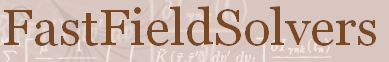FastFieldSolvers Forum
Home | Profile | Register | Active Topics | Members | Search | FAQ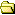All Forums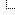FastFieldSolvers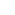FasterCap and FastCap2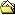2D Simulation - A query on Maxwell Capacitance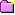New Topic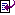Reply to Topic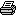Printer Friendly
Author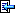Topic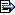Austria
9 Posts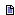Posted - Sep 23 2021 :  16:48:14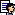Hi,first of all thanks to the FastFieldSolvers Team for making this software available to us!I am trying to see if I can benefit from the 2D capabilities of FasterCap, so I am poking around with simple structures to try and understand the fundamentals of the tool.In this phase, I am really hoping to leverage the (simpler) framework of a 2D simulation.I have been experimenting with a model for a parallel plates capacitor and I would have a few questions on it, but I'll leave that for later as here I would like to understand more about the capacitance matrix.For a structure with only two conductors, FasterCap returns a 1x1 matrix, as I would expect: just the cap between the two conductors I created.For a structure with two conductors over a gnd plane (i.e. a segment of conductor of larger size than the two conductors above it), the output is a 2x2 matrix.Now, I am referencing Enrico's white paper here: ht*ps://w*w.fastfieldsolvers.com/Papers/The_Maxwell_Capacitance_Matrix_WP110301_R02.pdfWhere the Maxwell Capacitance Matrix is explained with a 4-conductors example. The features I extract from the picture are:1. Each conductor has a 'self capacitance', i.e. a capacitance with respect to infinity2. Each conductor has N-1 (=3 in this case) mutual capacitances with all other conductors in the geometry.I have a little issue with this 'self capacitance', why it's needed, why it's there, so maybe that's the issue here.However, if I apply this scheme for a situation where I have two conductors, I would say that each conductor should have, again,1. A self capacitance (towards infinity)2. A mutual capacitance towards the other conductorTherefore, a 2x2 matrix. However, the output of FasterCap is a 1x1 matrix.Similarly, for a 2 conductors + gnd plane, you have 3 conductors (also gnd is a conductor, right?), so again if I follow the pattern explained in the white paper, I would expect a 3x3 matrix, not 2x2.What is the rationale behind this 'dimensional reduction'? Or maybe it's my input file incorrect?Thanks for helping!MicheleHere the file, for reference:(last version, two conductors and gnd plane)* 2D - Cap of two metal rods on a gnd surface --> 2x2 matrix?** data is included in a single file** Natural units are metres: how does cap scale?*C rectangle.txt 1 -6 0.0C rectangle.txt 1 5 0.0C gnd.txt 1 0 -10File rectangle.txt0 conductor strip*S microstrip 0.0 0.0 0.0 1.0S microstrip 0.0 1.0 1.0 1.0S microstrip 1.0 1.0 1.0 0.0S microstrip 1.0 0.0 0.0 0.0EndFile gnd.txt0 gnd plane*S gnd -10 0.0 10 0.0End

447 PostsPosted - Sep 27 2021 :  18:14:50Dear Michele,the explanation lies in the 2D capacitance case vs. 3D.In general, from theory, the potential is only defined up to an additive constant. When solving an electrostatic problem the value of the constant can be calculated from the boundary conditions.In the 3D case, since the potential goes as 1/r, where r is the distance of the observation point from the source, the potential at infinity would be 'k', where k is the constant. As boundary condition it is then customary to set the potential at infinity as zero; in this way, the constant is set to zero. The discussion then proceeds exactly as outlined in the Maxwell Capacitance Matrix white paper.In the 2D case, the potential goes as log(1/r). It is clear then, mathematically, that the potential at infinity does NOT go to zero. Seen in another, more intuitive way, you need to consider that the 2D case is not a zero-depth filament lying in a 3D space, but actually an infinitely long structure with constant cross-section corresponding to the 2D geometry. Therefore, the potential at infinity cannot be zero, as the structure itself extends to infinity. So, instead of explicitly calculating the additive constant, we can use a 'trick'. We consider only the voltage difference between the conductors, and not their absolute potentials (note that this decreases the system of equations from N to N-1; and to solve the system we need impose an additional constraint, in practice an energy boundary condition, requiring that the sum of all charges is zero).You should now appreciate why in the 2D case you do not have the capacitance to infinity but in fact only the capacitance to the other conductors. Note also, with respect to capacitance to infinity, that in 3D this is the capacitance of a single conductor; e.g. a sphere in the free space from Gauss theorem has a self-capacitance of 4*pi*e0 (where 'pi' is pi-greek and 'e0' is the vacuum permittivity).If you want a more rigorous treatement of the topic you can refer to J. Venkataraman, S. M. Rao, A. R. Djordjevic, T. K. Sarkar, "Analysis of Arbitrarily Oriented Microstrip Transmission Lines in Arbitrarily Shaped Dielectric Media Over a Finite Ground Plane", IEEE Transactions on Microwave Theory and Techniques, Vol. 33, No. 10, Oct 1985.For the relationship of the capacitance extraction of 2D geometries to transmission lines I can also suggest C. Wei, R. F. Harrington, J. R. Mautz, T. K. Sarkar, "Multiconductor transmission lines in multilayered dielectric media", IEEE Transactions on Microwave Theory and Techniques, Vol. 32, No. 4, Apr 1985.Regarding your question in the input file comments, i.e. "Natural units are metres: how does cap scale?", this again is different in the 2D and in the 3D case.In 3D, capacitance scales linearly with the dimensions. This is the reason why you don't need to specify dimensions in the FastCap or FasterCap input files. If your dimensions are meters, i.e. 1.0 in your file means 1m, your results will be in Farads. If your dimensions are mm, i.e. 1.0 in your file means 1mm, your results will be in milliFarads. If you want to automatically scale the results (e.g. see the results always in Farads) you can simply keep into account the scale in the eps_r constant you specify when running the simulation.In 2D instead the resulting capacitance is always a capacitance per unit length, i.e. F/m. Therefore if you re-scale your structure to be in mm, and read the results as F/mm, you will get exactly the same number (because the capacitance will scale linearly 10^-3 and the unit length as well).Best Regards,EnricoAustria
9 PostsPosted - Sep 27 2021 :  19:54:42Dear Enrico,many thanks for your explanation and references, I'll look them up!In the few days from my post to now, I think I got to something similar reasoning by myself, although I couldn't figure out the log(1/r) dependency on my own ;-)If I understand you right, then, the 2x2 matrix obtained from my arrangement, in 2D, is of the form:Q1 = c11*Vd1 + c12*Vd2Q2 = c21*Vd1 + c22*Vd2with the 'implicit' constraint that Q1+Q2=-Q3and Vd1 and Vd2 being deltas among the three conductors' voltages.Is this correct? If so, I would then ask: how are Vd1 and Vd2 defined?Meaning:1. What in the file determines them?2. How does the simulator communicate the choice, in its output?For the file I posted in the OP, the results of the matrix are:g1_microstrip 1.48869e-011 -4.3045e-012 g2_microstrip -4.31433e-012 1.48527e-011Could you explain that the 'gx_microstrip' label means?Thanks again!Michele447 PostsPosted - Sep 28 2021 :  15:51:33Hi Michele,I think I see your point - you are wondering where's the voltage of the third conductor. You're absolutely right in asking, as I'm afraid we kept it implicit i.e. it is not documented (actually it appears as a comment in the source code, but of course I don't expect anyone to browse the sources just to use the software..).In fact, in the 2D simulation one conductor is always considered ground, meaning that its potential is never raised to 1V. The voltage of this conductor is the famous one that is subtracted from the other to achieve the 'trick'. Now, FasterCap simply assumes that the last conductor that is defined in a list file is the ground conductor (I believe this is the missing piece of information you needed). If you check the 2D input file examples that are installed with the software, you will see in fact that the gnd definition always comes last.So in a nutshell, the Maxwell capacitance matrix you see for your 3 conductor example file refers to the first two conductors vs. the gnd plane (that you defined correctly as last conductor). So the C11 element is the sum of the capacitance per unit length to the second conductor and to the gnd plane and the C12=C21 element is the capacitance per unit length between the two conductors. Note by the way that this definition of the Maxwell capacitance matrix allows to invert the matrix to find also the inductance per unit length of the transmission line, if you assume TEM propagation (for the rigorous treatment again see C. Wei, R. F. Harrington, J. R. Mautz, T. K. Sarkar, "Multiconductor transmission lines in multilayered dielectric media", IEEE Transactions on Microwave Theory and Techniques, Vol. 32, No. 4, Apr 1985, that contains the proof).For what regards instead the g1_ and g2_ prefixes, this is not related to 2D or 3D extraction. In fact, these are prefixes that are added to the conductor name when you use the same conductor definition multiple times, to distinguish between the conductors. In your case you are using the rectangle.txt file twice, and the conductor name in the file is always 'microstrip'. To avoid shorting the two conductors together unintentionally, the name is prefixed by an incremental g_ string, where 'g' means 'group' and is simply a number.If you want specific names, you need to use files containing different names.Finally, let me just add a small remark about the sum of the charges; what you say is correct if you have no dielectric. In presence of a dielectric, you need to zero the sum of the total charges, i.e. including also the polarization charges at all interfaces. This is not (only) the free charge at the conductor surface; but this is a detail you can safely ignore, as it is not impacting at all your results or how you will use them.Hope this clarifies a bit,EnricoAustria
9 PostsPosted - Sep 28 2021 :  19:33:44Hi Enrico,many thanks for your detailed explanation. It makes perfect sense now!In the mean time, I gathered some other questions on my journey with the tool, but I'll start a new thread for those.Thanks!MicheleTopicNew TopicReply to TopicPrinter Friendly Jump To: Select Forum FastFieldSolvers       FastModel       FastImp       FastHenry2       FasterCap and FastCap2       E.M. Workbench       Development  -------------------- Home Active Topics Frequently Asked Questions Member Information Search Page
 FastFieldSolvers Forum © 2020 FastFieldSolvers S.R.L.